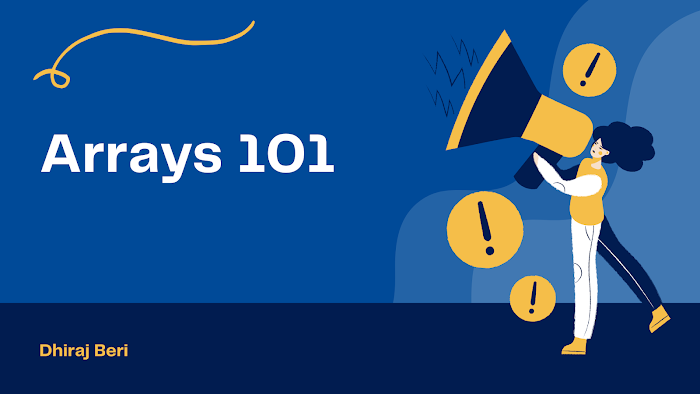Now it's time to move on from the array representation and learn some of its abstract data type operations. In simple words, we'll see how arrays work!

I will make it simple and complete into one single program, I create functions for each operation. This program updates in the future too!

In this program, I will explain Append, Insert, Delete, Linear Search, Improve Linear Search, Binary Search, and many more!

I explain each line in the comments.

`#include <stdio.h>#include <stdlib.h>struct Array{    int A;    int size;    int length;};//modify that's why the call by reference and x is number we want to appendvoid Append(struct Array *arr, int x){    if (arr->length < arr->size) //if length is less than size    {        arr->A[arr->length++] = x; //x will be added to array    }}void Insert(struct Array *arr, int index, int x){    int i;    if (index >= 0 && index <= arr->length) //index is valid    {        for (i = arr->length; i > index; i--)        {            arr->A[i] = arr->A[i - 1]; //previous element copy        }        arr->A[index] = x; //x will be added to index        //length will be increment because new element is added        arr->length++;    }}int Delete(struct Array *arr, int index){    int x = 0, i; //deleted value in x    if (index >= 0 && index < arr->length) //valid index    {        x = arr->A[index]; //x is assigned to index value        for (i = index; i < arr->length - 1; i++) //shifting the elements        {            arr->A[i] = arr->A[i + 1]; //copy previous element        }        arr->length--; //length decrement because one value is deleted        return x; //return deleted element    }    return 0;}//not modify the array that's why call by valueint LinearSearch(struct Array arr, int key){    int i;    for (i = 0; i < arr.length; i++) //saare value ke liye check karega    {        if (key == arr.A[i]) //agar key value ke uper toh index return        {            return i;        }    }    return -1;}void swap(int *x, int *y){    int temp;    temp = *x;    *x = *y;    *y = temp;}//Improve linear search me ya toh swap krke ek baar aage lao//ya fir direct first pe le aao//Next time jab bhi hum same element search kare toh time kam lage//Transpositionvoid ImproveLinearSearch1(struct Array *arr, int key){    int i;    for (i = 0; i < arr->length; i++)    {        if (key == arr->A[i])        {            swap(arr->A[i], arr->A[i - 1]); //swap a[i] and a[i-1]            return i;        }    }    return -1;}//Move to headvoid ImproveLinearSearch2(struct Array *arr, int key){    int i;    for (i = 0; i < arr->length; i++)    {        if (key == arr->A[i])        {            swap(arr->A[i], arr->A); //swap a[i] with first element            return i;        }    }    return -1;}int BinarySearch(struct Array arr, int key){    int l, mid, h;    l = 0;    h = arr.length - 1;    while (l <= h)    {        mid = (l + h) / 2;        if (key == arr.A[mid])            return mid;        else if (key < arr.A[mid])            h = mid;        else            l = mid + 1;    }    return -1;}int Get(struct Array arr, int index){    if (index >= 0 && index < arr.length)        return arr.A[index];    return -1;}void Set(struct Array *arr, int index, int x){    if (index >= 0 && index < arr->length)        arr->A[index] = x;}int Max(struct Array arr){    int max = arr.A;    int i;    for (i = 0; i < arr.length; i++)    {        if (arr.A[i] > max)        {            max = arr.A[i];        }    }    return max;}int Min(struct Array arr){    int min = arr.A;    int i;    for (i = 0; i < arr.length; i++)    {        if (arr.A[i] < min)        {            min = arr.A[i];        }    }    return min;}int Sum(struct Array arr){    int sum = 0;    int i;    for (i = 0; i < arr.length; i++)    {        sum = sum + arr.A[i];    }    return sum;}float Avg(struct Array arr){    return (float)Sum(arr) / arr.length;}// Reversing the Arrayvoid Reverse(struct Array *arr){    int *B; //new array    int i, j;    B = (int *)malloc(arr->length * sizeof(int)); //array ko heap me banaya    for (i = arr->length - 1, j = 0; i >= 0; i--, j++)    //last element se traverse hoga first array aur second array first element se        B[j] = arr->A[i]; //second array me copy ho jayga    for (i = 0; i < arr->length; i++)        arr->A[i] = B[i]; //seond array ki jarurat nahi hai toh first me copy kr diya vapas}//Swap first element with last element, second element with last second element aur aise krte jaana hai jab tak i<jvoid Reverse2(struct Array *arr){    int i, j;    for (i = 0, j = arr->length - 1; i < j; i++, j--)    {        swap(&arr->A[i], &arr->A[j]);    }}void Display(struct Array arr){    int i;    printf("Elements are: \n");    for (i = 0; i < arr.length; i++)    {        printf("%d ", arr.A[i]);    }}int main(){    struct Array arr = {{2, 3, 4, 5, 6}, 10, 5}; //{{elements}, size, length}    Append(&arr, 10);    Insert(&arr, 0, 1);    printf("Deleted element is: %d\n", Delete(&arr, 2));    Display(arr);    return 0;}`

Congratulations to you, if you really understand this program. Any doubts? Then you can ask me in the comment section.

As we covered array, this is the right time to solve some Leetcode easy array questions.

What's next?
Solved practice questions

After practicing, you are ready for the next data structure - Strings.